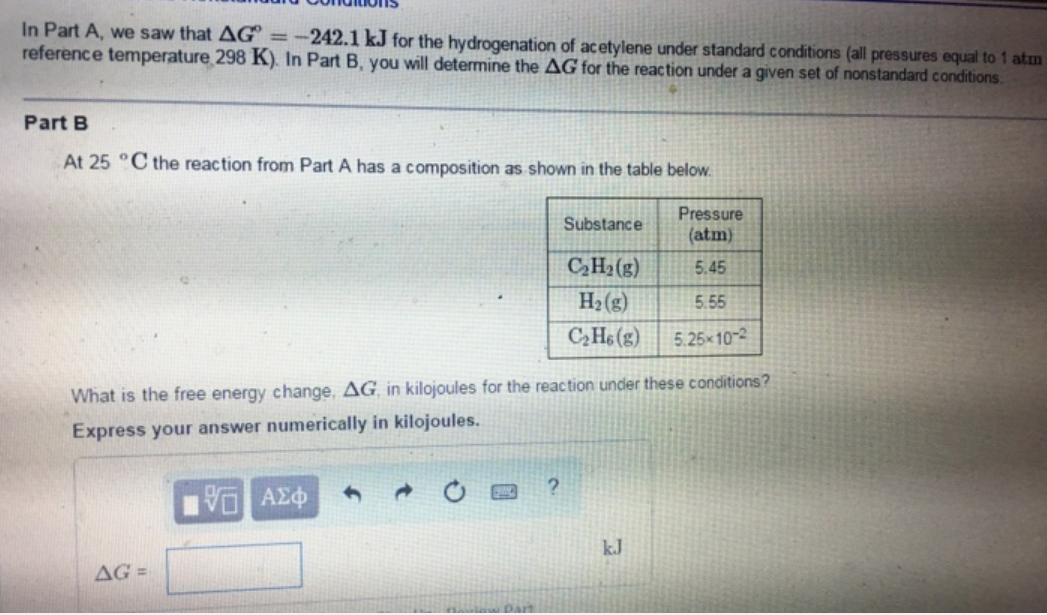# Problem: In Part A, we saw that delta G° = 242.1 kJ for the hydrogenation of acetylene under standard conditions (all pressures equal to 1 atm reference temperature 298 K). In Part B you will determine the delta G for the reaction under a given set of nonstandard conditions. At 25 ºC the reaction from Part A has a composition as shown in the table below. What is the free energy change, delta G, in kilojoules for the reaction under these conations? Express your answer numerically in kilojoules.

###### FREE Expert Solution
99% (165 ratings)###### Problem Details

In Part A, we saw that delta G° = 242.1 kJ for the hydrogenation of acetylene under standard conditions (all pressures equal to 1 atm reference temperature 298 K). In Part B you will determine the delta G for the reaction under a given set of nonstandard conditions. At 25 ºC the reaction from Part A has a composition as shown in the table below. What is the free energy change, delta G, in kilojoules for the reaction under these conations? Express your answer numerically in kilojoules.